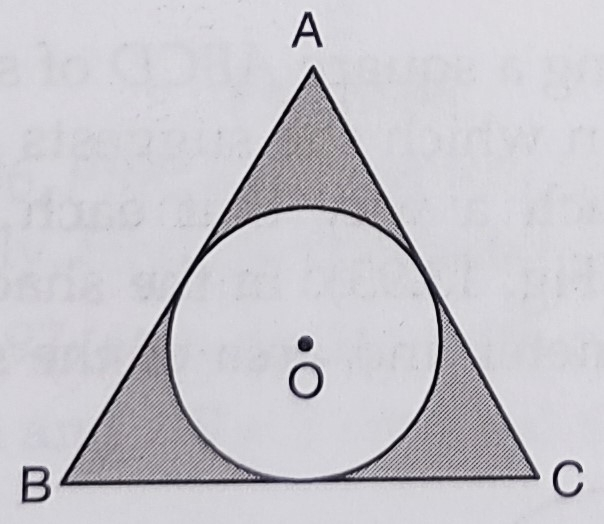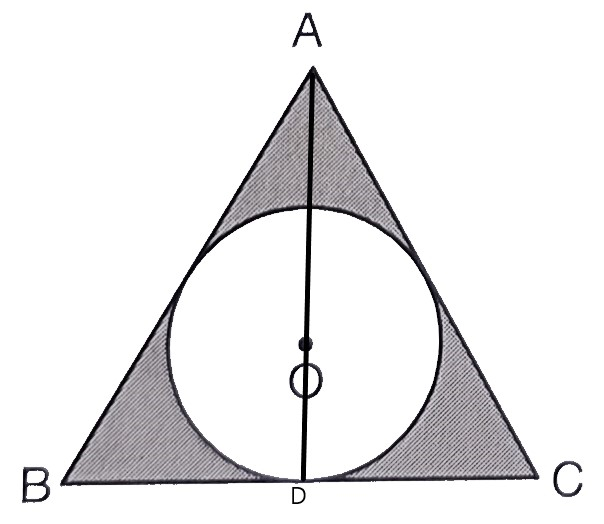"
">

# A circle is inscribed in an equilateral triangle $A B C$ is side $12 \mathrm{~cm}$, touching its sides. Find the radius of the inscribed circle and the area of the shaded part."

Given:

A circle is inscribed in an equilateral triangle $A B C$ of side $12 \mathrm{~cm}$, touching its sides.

To do:

We have to find the radius of the inscribed circle and the area of the shaded part.

Solution:

Length of each side of the equilateral triangle $ABC (a)= 12\ cm$

This implies,

Area of the equilateral triangle $=\frac{\sqrt{3}}{4} a^{2}$

$=\frac{\sqrt{3}}{4}(12)^{2}$

$=\frac{1.732 \times 12 \times 12}{4}$

$=62.352 \mathrm{~cm}^{2}$Draw $AD \perp BC$.

This implies,

$OD=\frac{1}{3} (AD)$                 [Since, $O$ is the centroid of the equilateral triangle]

$=\frac{1}{3} \times \frac{\sqrt{3}}{2} \times 12$

$=\frac{\sqrt{3}}{6} \times 12$

$=2 \sqrt{3}$

Radius of the incircle $r=OD$

$=2 \sqrt{3} \mathrm{~cm}$

Area of incircle $=\pi r^{2}$

$=\frac{22}{7} \times(2 \sqrt{3})^{2}$

$=\frac{22}{7} \times 12$

$=\frac{264}{7}$

$=37.714 \mathrm{~cm}^{2}$

Therefore,

Area of the shaded region $=62.352-37.714$

$=24.638 \mathrm{~cm}^{2}$

The radius of the inscribed circle is $2\sqrt3\ cm$ and the area of the shaded part is $24.638\ cm^2$.

Updated on: 10-Oct-2022

108 Views